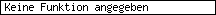# What happens when pcl3 is heated

authormessage
???
guestPosted: March 26, 2016 8:58 am Title: QuestionsMy question: hello please for your help 1.) If a sample of 1.50 mol of PCl5 is heated in a liter flask to 280 degrees Celsius, an equilibrium is established: PCl5-> PCl3 + Cl2 KC at this temperature is 2 mol / l Find all equilibrium concentrations for all compounds 2.) From one liter of an aqueous solution containing 0.1 mol Cu2 + and 0.1 molAg + ions, the copper ions should be precipitated by introducing hydrogen sulfide up to a residual concentration of 10 ^ -8 mol / l. Calculate the concentration of silver ions in the solution after sulfide precipitation and the mass of precipitated silver sulfide (PkL (CUS) = 44 PKL (Ag2S) = 50 relative atomic masses: Ag = 108 S: 32) Many Thanks My ideas: to 1.) C = n * V C = 1.5 * 1 = 1.5 mol / l for PCl5 2 = (X * X) / (1.50 mol / l) thus KC = concentration of the products by starting materials x ^ 2 / (1.5) = 2 x ^ 2 = 3 x1 = root3 x2 = root 3 The concentration for PC3 and Cl2 is root3 mol / l to 2.) I have no idea, sorrymagician4Registration date: 05.10.2009
Posts: 11677
Place of residence: HamburgPosted: March 26, 2016 12:28 PM Subject:Quote: to 1.) C = n * V C = 1.5 * 1 = 1.5 mol / l for PCl5

No:and therefore, in a 1-L flask:Quote: 2 = (X * X) / (1.50 mol / l)

No:
from your conversion equation (11 + 1) the result is that if you go from the initial state (you only have c0(PCl5)) to the equilibrium state with cGG(PCl5), cGG(PCl3) and cGG(Cl2), then for every x mol of PCl3 , Cl2 exactly also x mol PCl5 be decomposed

therefore:with the chemically plausible of the two mathematically valid solutions:and thus, using (a):

cGG(PCl5) = 1.5 mol / L - 1 mol / L = 0.5 mol / L
cGG(PCl3) = cGG(Cl2) = 0 + 1 mol / L = 1 mol / L

to (2)
this is about the so-called "solubility product"
for an A1B.1 salt (and this is what CuS is about) is thisfor an A2B.1 salt (and Ag2S) is thissoderle, 1st step:
you should in your sauce H2Introduce S until the remaining concentration of Cu2+ only 10-8 mol / L. *)this residual copper ion concentration then fulfills the solubility product according to formalism (a)

... and thus:the sulfide ion concentration at this point in time is 10-32 minor

2nd step: when the sulphide ion concentration has reached this value, it was already the L beforehandp of the Ag2S had been exceeded, the Ag+-ions already massively "emaciated". the value that they are down to results from the sulphide concentration calculated above according to formalism (b):

[Image_Link]http://www.matheboard.de/latex2png/latex2png.php?K_L(Ag_2S) = 10^{-pKL} = 10^{-50} mol^3/L^3 = [Ag^+]^2_{grenz} * [S^{2-}]_{(ende CuS-faellung)} \\
= [Ag^+]^2_{grenz} * 10^{-32} mol/L [/Image_Link]measured by the initial concentration of [Ag+] of 0.1 mol / L this corresponds to an excellent approximation of a quantitative fall. **)
You can therefore argue that the previously present silver ions (that was 0.1 mol, absolute in one liter) then half of this amount of substance, i.e. 0.05 mol of Ag2S () have generated (because in 1 Ag2S are 2 Ag+)
the M of Ag2However, S is (108 + 108 + 32) g / mol = 248 g / mol, so that 0.05 mol of it then has a mass of 12.4 gthat is the mass of your parallel precipitated silver sulphide when your sulphide concentration finally drops to 10-32 mol / L is what you had to achieve in order to reduce the copper ions to the specified / required concentration.

greeting

Ingo

*)
Of course, before this state is reached, CuS already fails en masse: however, this is of no particular importance for this calculation, since we only need to be interested in the (specified) final concentration of copper ions to be achieved.

of course it is already Ag2S failed: we will take care of that in the 2nd step

**)
you can of course do the math more precisely: 0.5 * (0.1 - 10-9) mol of silver sulfide have precipitated
... but before the approx. forty-second decimal place nothing else comes out than the above approximationcompletely banana
_________________
a month in the laboratory saves you a quarter of an hour in the library!